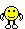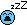Discussion about math, puzzles, games and fun.   Useful symbols: ÷ × ½ √ ∞ ≠ ≤ ≥ ≈ ⇒ ± ∈ Δ θ ∴ ∑ ∫  π  -¹ ² ³ °

You are not logged in.

## #1 2012-12-04 09:24:17

gbrad88
Member
Registered: 2012-12-03
Posts: 16

### Condense the logorithmic expression

Condense the logorithmic expression as much as possible:

3 ln x -(1/3) ln y

The answer is  ln x^3 / 3(radical)y

Can a process be provided as to how one gets to the answer? I think I see what is happening, but could use clarification.

Offline

## #2 2012-12-04 10:24:57

anonimnystefy
Real MemberFrom: Harlan's World
Registered: 2011-05-23
Posts: 16,039

### Re: Condense the logorithmic expression

Try using this formula, first:

Tell me what you get after applying the formula.

Here lies the reader who will never open this book. He is forever dead.
Taking a new step, uttering a new word, is what people fear most. ― Fyodor Dostoyevsky, Crime and Punishment
The knowledge of some things as a function of age is a delta function.

Offline

## #3 2012-12-04 18:11:57

noelevans
Member
Registered: 2012-07-20
Posts: 236

### Re: Condense the logorithmic expression

Hi!I hope you have had a chance to try stefy's suggestion and solved the problem.  If not, then
give it a try before looking at the following proof of the rule involved in solving the problem.

The definition of log is usually introduced via two equalities in two variables, namely,
x
N = 10    and   x = logN which somewhat obscures the meaning of the logarithm.

logN
If we substitute logN for x in the first equality we get  N = 10        ("lay on goodies" to get N.)
which gives us a single equality with a single variable.  And from this single equality we can
basically read the definition of logN:  "logN is the exponent we must apply to 10 to get N."
logN is a NAME for the right exponent that applied to 10 produces N.  So logs are names for
exponents.  And the naming is nice in that it tells us what the base is that it must be applied to
and the result we get when we apply it to that base.  If the base is b then the logarithm is
log N
b
log  N which when applied to base b results in N; that is,  b        = N.
b

(In Maple the log is written log[b](N).)

logN
Writing N as 10       (by the definition of log) we obtain
p
p       logN p        p*logN                                                p        log(N  )
N  =(10      )  =  10           by a law of exponents.    Also  N   = 10          by the definition of log.
p                                                                      p
( x   was immediately above but should have been N   left of the equal sign.  That's the edit.)
p
p*logN        log(N  )
Thus  10          = 10             and since the bases are equal and the quantities are equal then
p
the exponents must be equal; that is,  p*logN = log(N  ).  (because log is a 1-1 function.)

p                                                                       p
More generally   p*log N = log (N  )  so the exponent on N in the argument of log (N  )
b         b                                                                       b
p
"comes out front" forming p*log N.   And if b=e then we have p*lnN = ln(N  )
b
n  m     nm                       logN  p       p*logN
This is a disguised form of the law of exponents:  (x  )   = x      .  Here it is (10      )   = 10        .

In the original problem the p=3 for x and the p=1/3 for the y.

Then the difference produces a quotient in the argument of the single logarithm.

3        1/3             3   1/3
3lnx-(1/3)lny =  lnx  - ln(y     )  =  ln(x /y     )

p
In base 10 the rule can be written:    plogN = logN .
n m      nm
The law of exponents is:  exponentiation produces a product   (N  )   = N     .
p
Similarly the exponentiation in log(N  )  produces the product P*logN.

The laws of logarithms are disguised forms of the laws of exponents because logarithms ARE
exponents.Hasta la vista!  Gotta get someLast edited by noelevans (2012-12-06 10:23:05)

Writing "pretty" math (two dimensional) is easier to read and grasp than LaTex (one dimensional).
LaTex is like painting on many strips of paper and then stacking them to see what picture they make.

Offline

## #4 2012-12-05 05:05:13

gbrad88
Member
Registered: 2012-12-03
Posts: 16

### Re: Condense the logorithmic expression

Whew, I don't know. This is the upper limit of my ability and understanding. This is my final unit in my semester and this was a very bad unit for me. Thankfully my grades were high enough up to this point, but Im really hitting the wall on understanding the log, ln, all of that.

Offline

## #5 2012-12-05 06:01:57

anonimnystefy
Real MemberFrom: Harlan's World
Registered: 2011-05-23
Posts: 16,039

### Re: Condense the logorithmic expression

Hi gbrad88

Have you read noelevans post (post #3 in this thread)?

Here lies the reader who will never open this book. He is forever dead.
Taking a new step, uttering a new word, is what people fear most. ― Fyodor Dostoyevsky, Crime and Punishment
The knowledge of some things as a function of age is a delta function.

Offline

## #6 2012-12-05 06:42:40

gbrad88
Member
Registered: 2012-12-03
Posts: 16

### Re: Condense the logorithmic expression

YES, and although it wants to make sense, its is bouncing between my ears unclear when I try to apply. Ive been trying to short cut the process to come close to an answer.

Offline

## #7 2012-12-05 06:55:04

bob bundy
AdministratorRegistered: 2010-06-20
Posts: 8,507

### Re: Condense the logorithmic expression

hi gbrad88,

This, and the other problems you have posted, can all be done using one or more of the following:

Bob

Children are not defined by school ...........The Fonz
You cannot teach a man anything;  you can only help him find it within himself..........Galileo Galilei
Sometimes I deliberately make mistakes, just to test you!  …………….Bob BundyOffline

## Board footer

Powered by FluxBB